Anzeige

# G7-Topic-1-SETS.pptx

30. Mar 2023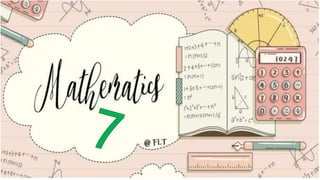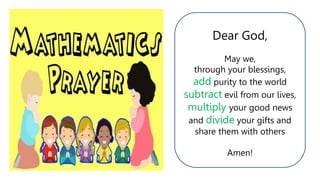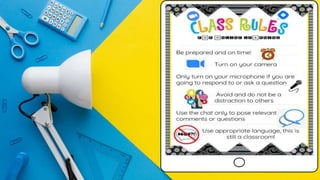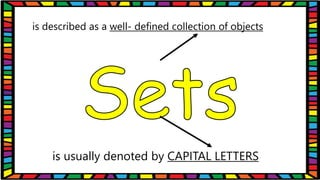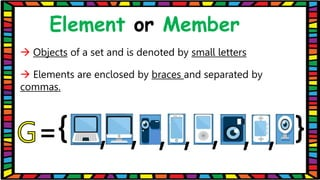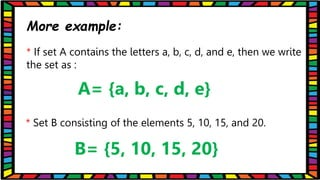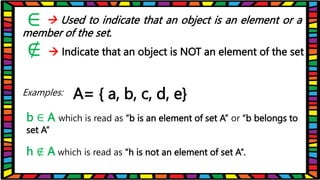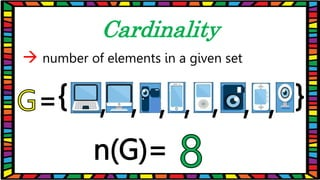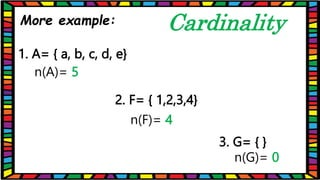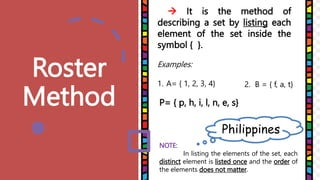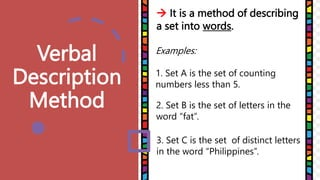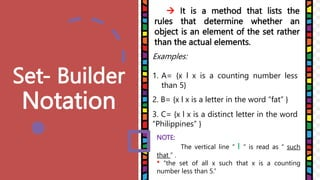1 von 21
Anzeige

### G7-Topic-1-SETS.pptx

1. Dear God, May we, through your blessings, add purity to the world subtract evil from our lives, multiply your good news and divide your gifts and share them with others Amen!
2. 3
4. A set of Sports Ball
5. A Flock of Birds
6. A Family of Plants
7. A Set of Utensils
8. is described as a well- defined collection of objects is usually denoted by CAPITAL LETTERS
9. Element or Member
10.  Objects of a set and is denoted by small letters  Elements are enclosed by braces and separated by commas. Element or Member
11. More example: * If set A contains the letters a, b, c, d, and e, then we write the set as : * Set B consisting of the elements 5, 10, 15, and 20. A= {a, b, c, d, e} B= {5, 10, 15, 20}
12.  Used to indicate that an object is an element or a member of the set. Examples: ∈  Indicate that an object is NOT an element of the set ∉ A= { a, b, c, d, e} b ∈ A which is read as “b is an element of set A” or “b belongs to set A” h ∉ A which is read as “h is not an element of set A”.
13.  number of elements in a given set Cardinality
14. 1. A= { a, b, c, d, e} n(A)= 5 2. F= { 1,2,3,4} n(F)= 4 3. G= { } n(G)= 0 Cardinality More example:
15. Roster Method  It is the method of describing a set by listing each element of the set inside the symbol { }. Examples: 1. A= { 1, 2, 3, 4} NOTE: In listing the elements of the set, each distinct element is listed once and the order of the elements does not matter. Philippines 2. B = { f, a, t} P= { p, h, i, l, n, e, s}
16. Verbal Description Method  It is a method of describing a set into words. Examples: 1. Set A is the set of counting numbers less than 5. 3. Set C is the set of distinct letters in the word “Philippines”. 2. Set B is the set of letters in the word “fat”.
17. Set- Builder Notation  It is a method that lists the rules that determine whether an object is an element of the set rather than the actual elements. Examples: 1. A= {x l x is a counting number less than 5} NOTE: The vertical line “ l “ is read as “ such that ” . * “the set of all x such that x is a counting number less than 5.” 2. B= {x l x is a letter in the word “fat” } 3. C= {x l x is a distinct letter in the word “Philippines” }
Anzeige